Courses

# Image Formation by Concave and Convex Mirror Class 10 Notes | EduRev

## Class 10 : Image Formation by Concave and Convex Mirror Class 10 Notes | EduRev

The document Image Formation by Concave and Convex Mirror Class 10 Notes | EduRev is a part of the Class 10 Course Science Class 10.
All you need of Class 10 at this link: Class 10

What is a Mirror?

A mirror is a reflective surface that bounces off light, producing either a real image or a virtual image. When an object is placed in front of a mirror, the image of the same object is seen in the mirror.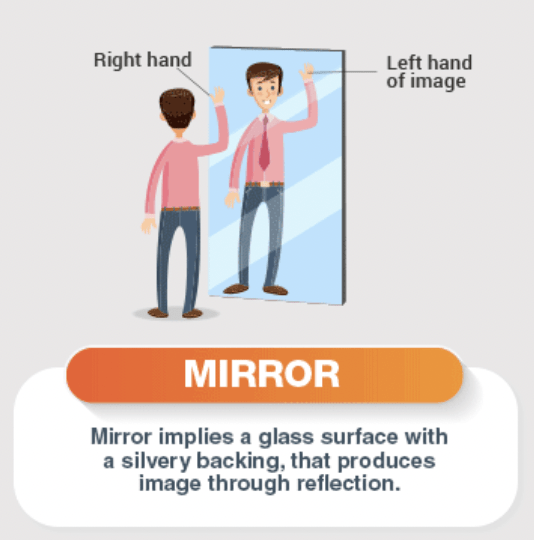• A smooth, highly polished reflecting surface is called a Mirror.
• A mirror bounces off light, producing either a real image or a virtual image. When an object is placed in front of a mirror, the image of the same object is seen in the mirror. The object is the source of the incident rays and the image is formed by the reflected rays.
• Based on the interaction of light, the images are classified as either a real image or a virtual image. A real image occurs when the light rays actually intersect while virtual images occur due to the apparent divergence of light rays from a point.

Types of Mirror

From the reflecting surface of the mirror there are two types of mirror:

• Plane mirror
• Spherical or curved mirror

Plane Mirror

• A highly polished plane surface is called a plane mirror or if a flat (totally plane) surface of a glass plate is polished one side with a reflecting material is called plane mirror.Formation of Image by a Plane Mirror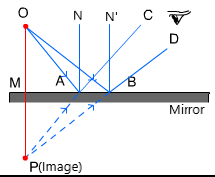• The image formed by a plane mirror is always virtual and erect.
• Object and image are equidistant from the mirror.

Properties of Image from flat (Plane) Mirror

• Virtual and erect.
• Same as the size of the object.
• The image is formed behind the mirror (as far as the object from the mirror).
• The image formed is laterally inverted.

Spherical Mirror

• A mirror whose polished, reflecting surface is a part of a hollow sphere of glass, is called a spherical mirror. For a spherical mirror, one of the two curved surfaces is coated with a thin layer of silver followed by a coating of red lead oxide paint. Thus, one side of the spherical mirror is made opaque and the other side acts as a reflecting surface.
• With respect to the polishing side there are two types of spherical mirrors: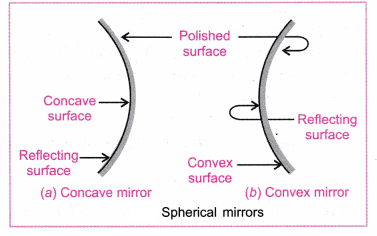(a) Convex (Diverging) Mirror: A spherical mirror whose outer bulging out surface is the reflecting surface.
(b) Concave (Converging) Mirror: A spherical mirror whose inner hollow surface is the reflecting surface.

Rules of Image Formation from the Spherical Mirror

The rules of reflection from the spherical mirror are based on incident and reflection angle.

1. A ray parallel to the principal axis after reflection from the mirror passes or appears to pass through its focus by definition of focus.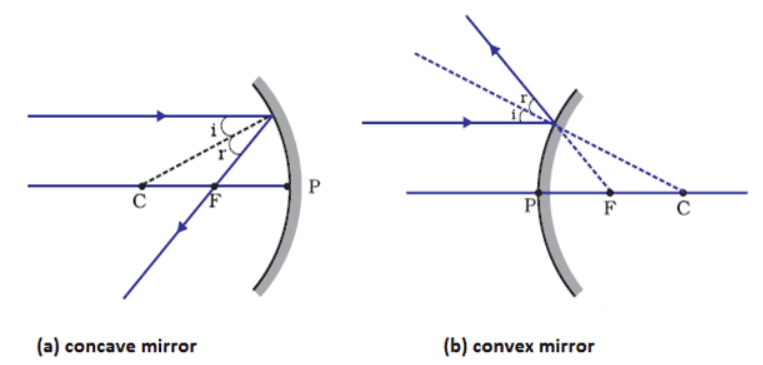2. A ray passing through or directed towards focus after reflection from the mirror will become parallel to the principal axis.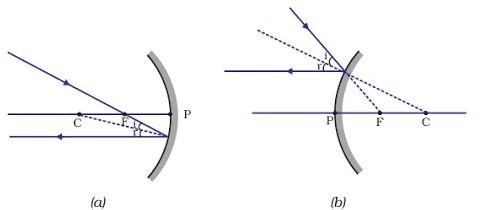3. A ray passing through or directed towards the centre of curvature after reflection from the mirror retraces its path. as for it ∠i = 0 and so ∠r = 0. It is because the light ray strikes the mirror at a right angle.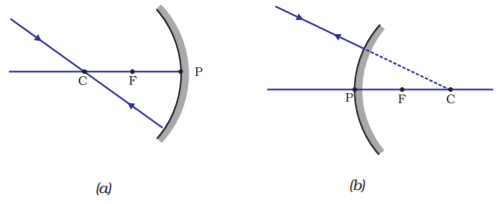4. Incident and reflected rays at the pole of a mirror are symmetrical about the principal axis.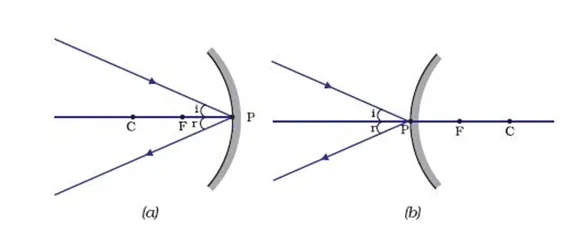Lateral Inversion and Inversion

• The phenomenon due to which the image of an object turns through an angle of 180° through vertical axis rather than horizontal axis, such that the right side of the image appears as left or vice versa is called lateral inversion.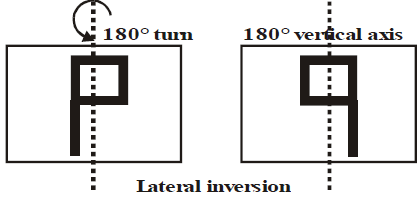• Inversion: During inversion, the image turns around horizontal axis through an angle of 180°.

Image Formation from Concave Mirror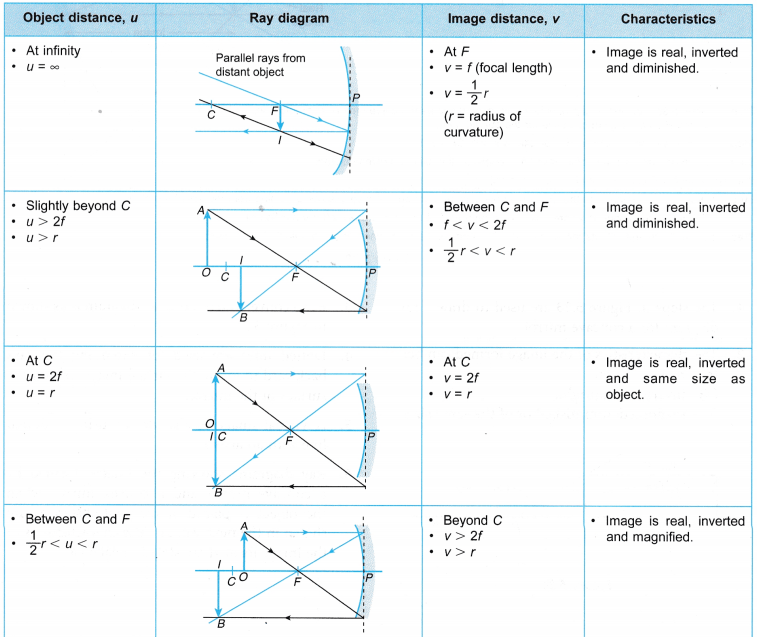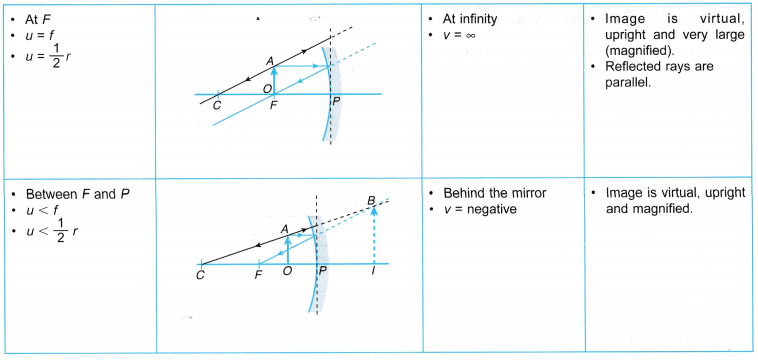Image Formation from Convex mirror

1. When the object is at infinity

• When the rays of light come (diverge) from an object situated at infinity, they are always parallel.  These parallel rays, strike the convex mirror and reflect to diverge outward from the convex mirror. These rays seem (appear) to come from focus.
• The characteristics of the image are virtual, erect, diminished to a point and formed at principal focus behind the convex mirror.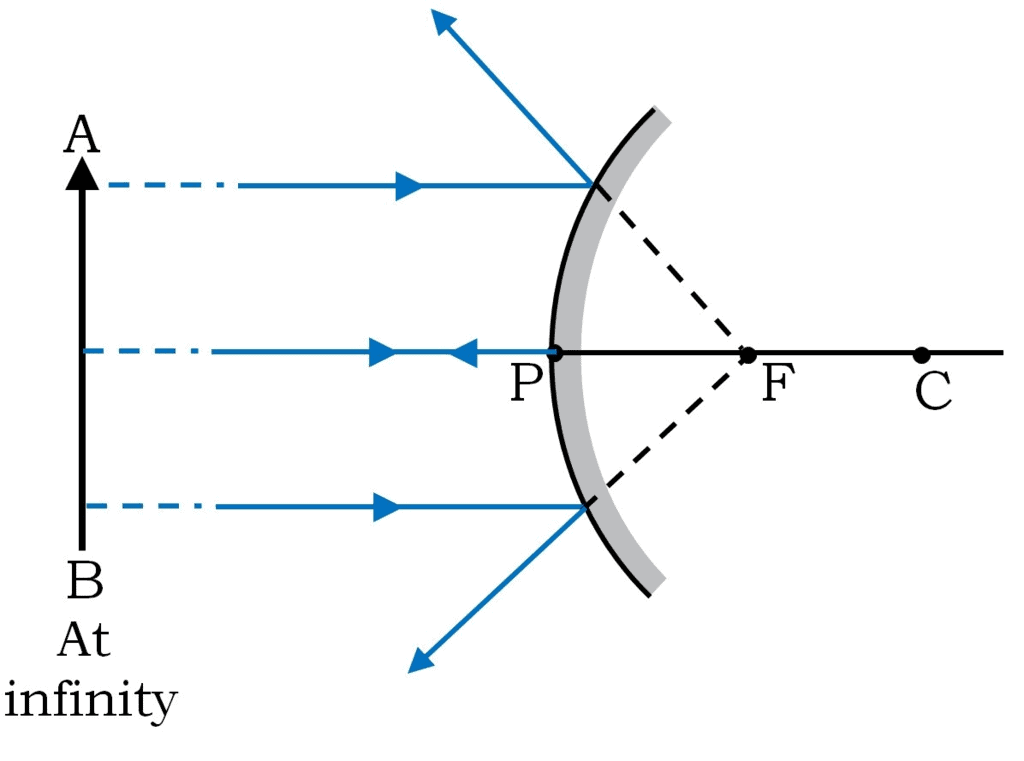2. When the object is at a finite distance from the Pole

When the image is formed between pole and principal focus behind the convex mirror, the image formed is virtual, erect and diminished.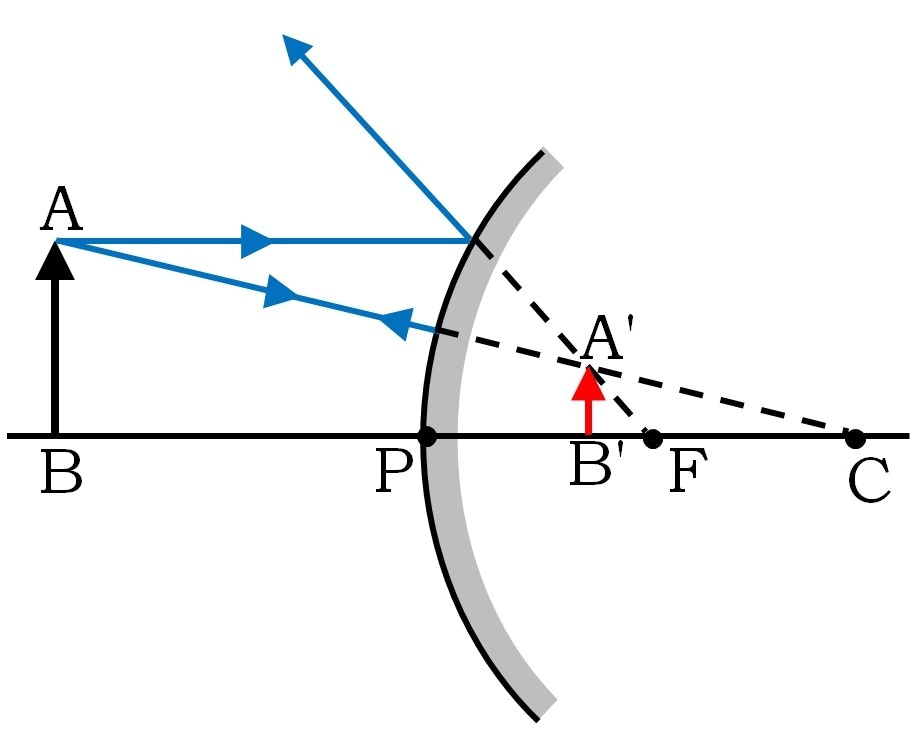Note:

There are only two positions of the object for showing the image formed by a convex mirror that is:

• When the object is at infinity.
• When the object is at a finite distance from the pole of the convex mirror. Beside these positions, no other positions are possible because the focus and the centre of curvature is behind the reflecting surface of the convex mirror.

Now we can study the image formation by following table: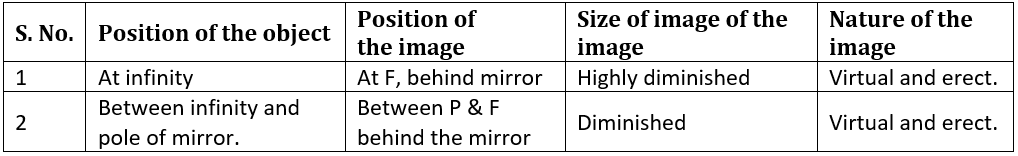Sign convention of Spherical mirror

• Whenever and wherever possible the ray of light is taken to travel from left to right.
• The distances above the principal axis are taken to be positive while below it is negative.
• Along the principal axis, distances are measured from the pole and in the direction of light are taken to be positive while opposite to it is negative.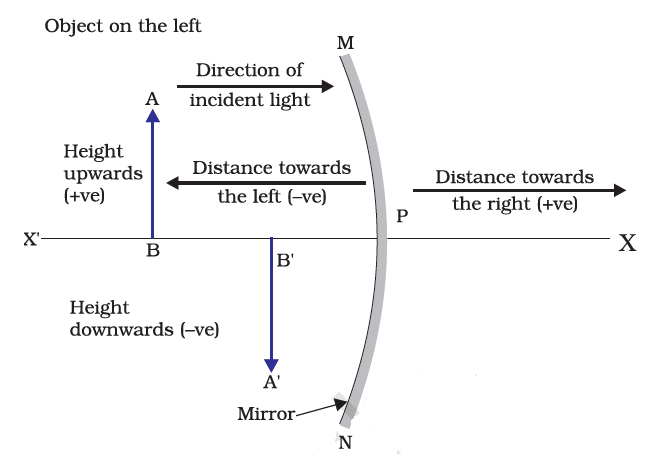Relation from Spherical mirror

1. Relation between f and R for the spherical mirror: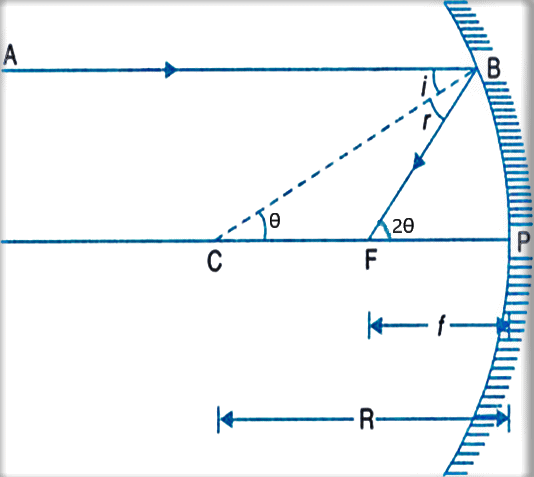If B is near line P

• Then from ΔBCP
tanθ ~ θ = BP/R
• From ΔBFP

tan2θ ~ 2θ = BP/f

• So, 2BP/R = BP/f
=> f = R/2

2. Relation between u,v and f for curved mirror: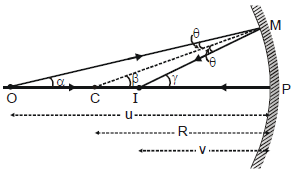• If an object is placed at a distance u from the pole of a mirror and its image is formed at a distance v (from the pole).
⇒ If angle is very small: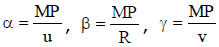⇒ from ΔCMO,
β = α + θ ⇒ θ = β - α
⇒ from ΔCMI,
γ = β + θ ⇒ θ = g - β
⇒ so we can write β - α = γ - β ⇒ 2β = γ + α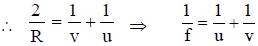Difference between Real and Imaginary Image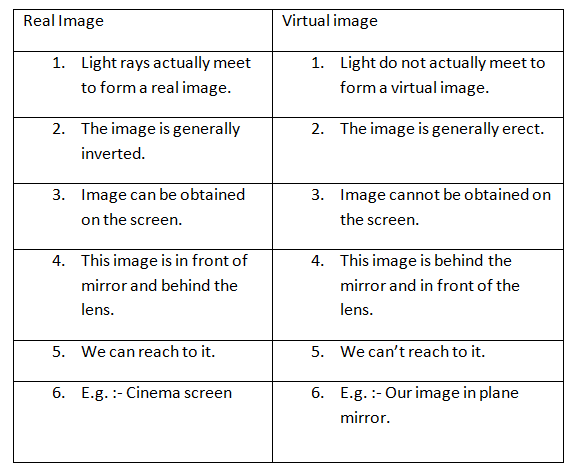Offer running on EduRev: Apply code STAYHOME200 to get INR 200 off on our premium plan EduRev Infinity!

## Science Class 10

80 videos|257 docs|114 tests

,

,

,

,

,

,

,

,

,

,

,

,

,

,

,

,

,

,

,

,

,

;